×#### Thank you for registering.

One of our academic counsellors will contact you within 1 working day.

Click to Chat

1800-1023-196

+91-120-4616500

CART 0

• 0

MY CART (5)

Use Coupon: CART20 and get 20% off on all online Study Material

ITEM
DETAILS
MRP
DISCOUNT
FINAL PRICE
Total Price: Rs.

There are no items in this cart.
Continue Shopping```Quadratic Equations

Table of Content

Who came up with the Quadratic Formula?

What is the Quadratic Equation and what is it used for?

How do you write a Quadratic Equation in standard form?

What actually the Degree of Polynomial means?

How to solve a Quadratic Equation?

Relation between Roots and Coefficient

Nature of Roots

What does it mean to have a Double Root?

What is a Real Solution?

Who came up with the Quadratic Formula?

Geometrical approach for finding out the solutions of quadratic equations was developed by Greek mathematician Euclid. Solving of quadratic equations, in general form, is often credited to ancient Indian Mathematicians. In fact, Brahmagupta (A.D.598–665) gave an explicit formula to solve a quadratic equation of the form ax2 + bx = c. Later, Sridharacharya (A.D. 1025) derived a formula, now known as the quadratic formula, (as quoted by Bhaskara II) for solving a quadratic equation by the method of completing the square.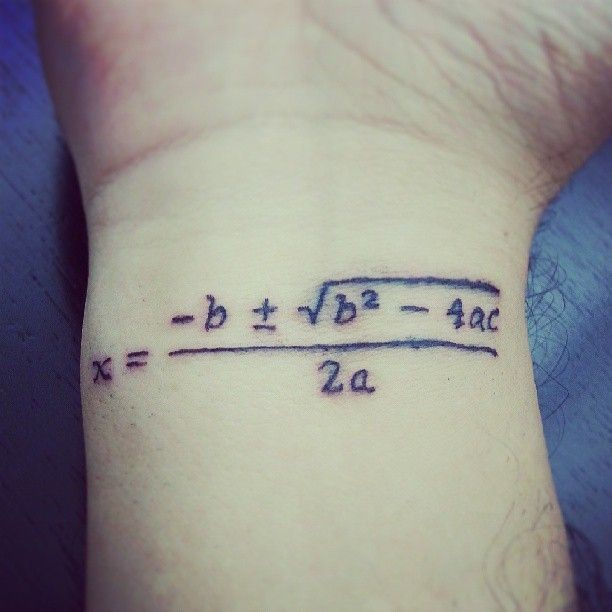What is the Quadratic Equation and what is it used for?

Quadratic Equation is one of the special case of polynomial equation. So before understanding quadratic, let’s first understand the concept of Polynomial Equation.

In Mathematics, Polynomial is an algebraic expression involving the variables and the coefficients.

The polynomial in single variable can be represented in general form as: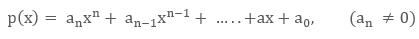Here, an, an-1, …, a, a0  are constants and called as the Coefficients of xn, xn-1, …., x, x0 respectively.

The powers of the variable x can only be natural numbers and the highest power in a polynomial is called as the degree.

So on the basis of the degree of any polynomial, they have been given special names.

Below table clearly explains this concept:

Degree of the polynomial
Name of the Polynomial

0
Zero Order or constant Polynomial

1
Linear Equation

2

3
Cubic Equation

4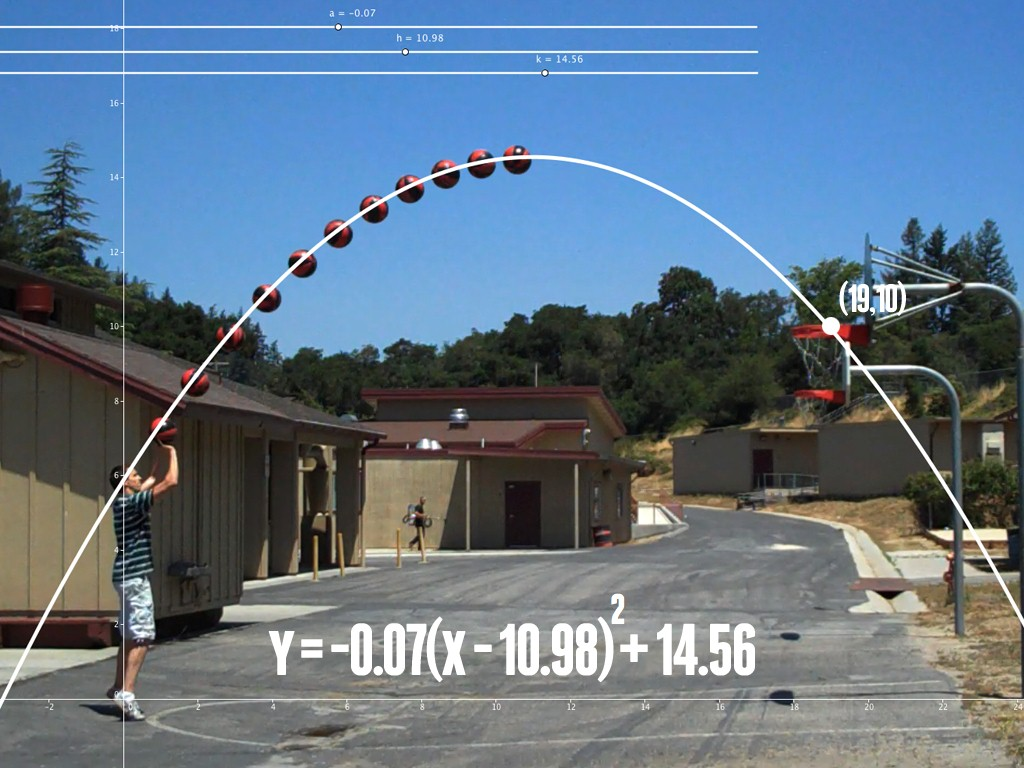How do you write a Quadratic Equation in standard form?

Any equation of the form  P(x) = 0, where p(x) is a polynomial having its degree equals to two is called as Quadratic Equation.

If this quadratic is arranged in the form where the terms are arranged in the decreasing order of their powers is called as its Standard Form.

So, the standard form of a quadratic equation is as:

ax2 +  bx + c = 0,  (a ≠0)

Where, a, b and c are called as the coefficients of x2, x and x0 respectively. c is also called as the Constant Term. In this equation, x is unknown quantity and therefore it is called as the Variable. A general quadratic equation consists of three terms ax2, bx and c.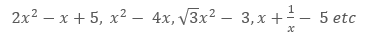What actually the Degree of Polynomial means?

Theoretically, the degree is the higher power in an algebraic expression.

Conceptually, the degree represents the number of possible solutions for an algebraic expression.

For example, for linear equation in one variable (degree = 1), we will have only one solution for that equation. While for quadratic equation in one variable (degree = 2), we will have two values of the variable satisfying the same equation. So in general, for nth degree polynomial, we will have n solutions for that equation.

Example 1   2x + 10 = 0 (Linear Equation, degree =1)

2x = -10

x = – 5 (Ons solution)

Example 2   x2 + 3x + 2 = 0 (Quadratic Equation, degree = 2)

x2 + x + 2x + 2 = 0

(x + 1) (x + 2) = 0

x = -1, -2 (Two solutions)

Graphically, Degree represents the number of points where the graph cuts the x – axis. So, for zero order or constant polynomial, the graph will be parallel and will not cut the x – axis for any value of x. For linear equation having degree = 1, we will have only one point where the graph will cut the x – axis. This point represents its solution. Similarly, for quadratic equation (degree = 2), their exists two points where the graph can cut/touch the x- axis at most.

Please see below for the graphs of different polynomials. In the graphs, n represents degree.Quadratic Expression is an expression p(x) having the degree two. While if quadratic expression is equated to zero that is p(x) is called as the Quadratic Equation.

For example: x2+ 8x+2 is quadratic expression. While x2 + 2x + 1 = 0 is quadratic equation as it also including equals to “= “ sign.

How to solve a Quadratic Equation?

Solving Quadratic equation means finding the values of the variable which satisfies the given equation.

Quadratic equation can be solved in two ways:

Factorization Method

Hindu Method or Sri dharachary method

Factorization Method: This method is based on the factor theorem, which says that if for any polynomial (x), for any x = k, if p(k) = Reminder = 0, then (x – k) is a factor of p(x).

Thus from given equation, we try to extract the factors which can easily leads to our solution. For quadratic equation, we can have maximum of two factors corresponding to two roots or solutions.

Example  3x2 - 2x – 1 = 0

3x2 - 3x + x -1 = 0

(x – 1) (3x + 1) = 0

x = 1, -1/3  are roots

Hindu Method: Here we use the method of completing the square method (studied in grade 10) to get the solution.

ax2 + bx + c = 0 (a ≠ 0, a, b, c ∈ R)

Divide above equation by a to make the coefficient of x2 to be unity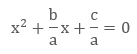Converting into perfect squareSo, here are the two roots for the quadratic equationHere, D is called as the Discriminant and its value is given by D = b2 – 4ac.

Relation between Roots and Coefficient

Assuming the α and β to be the roots of general equation, ax2 + bx + c = 0

Then by factor theorem, we can write the above equation as,

ax2 + bx + c = a(x- α) (x- β)

ax2 + bx + c =ax2-a(α + β)x + aαβ

On comparing the coefficients, we getAnd on further simplification, we get aFrom this we may also conclude that, if we are given the two roots then with the help of sum of roots that is α + β  and the product of roots that is αβ we can get the original equation as:

x2- x(α + β) + αβ = 0

Note: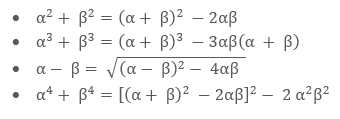Nature of Roots

The value of D =

Nature of Roots

The value of D =  b2 - 4ac in quadratic means discriminant. Discriminant plays most important role in deciding the nature of the roots. It is also represented by delta or ∆.

The roots are given by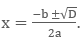So depending upon the nature of D, we may get repeated, distinct or complex solutions.If D = 0, means equal roots. Graphically, parabola touches the x-axis at a single point.

If D > 0, means real and distinct roots. Graphically, parabola intersects the x-axis at two distinct points.

And if D < 0, means non-real or imaginary or complex roots. In this case the parabola never touches the x-axis.

Note:

If co-efficients are rational then its irrational roots always occur in pair that is

p+ √q   and  p- √q.

If co-efficients are imaginary then its roots are always complex conjugate i.e. if one root is a + ib then other root is a - ib.

What does it mean to have a Double Root?

If discriminant value equals to zero that is D = 0 then from the equation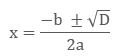We have,

x = -b/2aSo both the roots will be same which means for the quadratic we only have one roots or both roots are same. In this condition, the equation is said to be having double root or repeated roots.

In this case, the parabolic graph of the quadratic touches (instead to intersecting) the x – axis or in other words we can say that x- axis acts as a tangent to the graph.

What is a Real Solution?

Real Solution means the roots of the quadratic are not complex. That is while solving the quadratic equation, we should get its roots as real number instead of complex. It may be either two distinct real solutions or double (or repeated) real solutions but not complex solutions.

For Real Solutions, the Discriminant value that is D or ∆ should be either equals to or greater than zero.

For Real Solutions, D or ∆ ≥ 0Graphically, the graph of equation either touches or cuts the x – axis.

```### Course Features

• 731 Video Lectures
• Revision Notes
• Previous Year Papers
• Mind Map
• Study Planner
• NCERT Solutions
• Discussion Forum
• Test paper with Video Solution